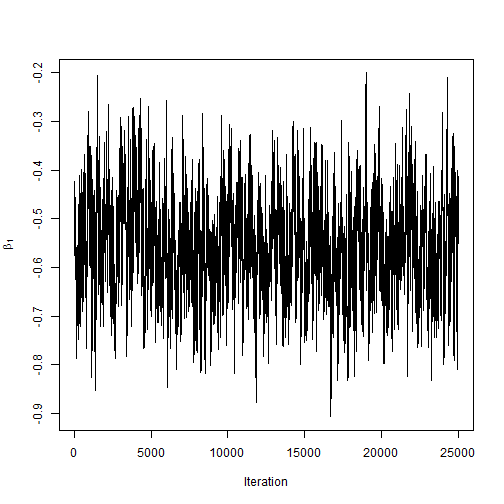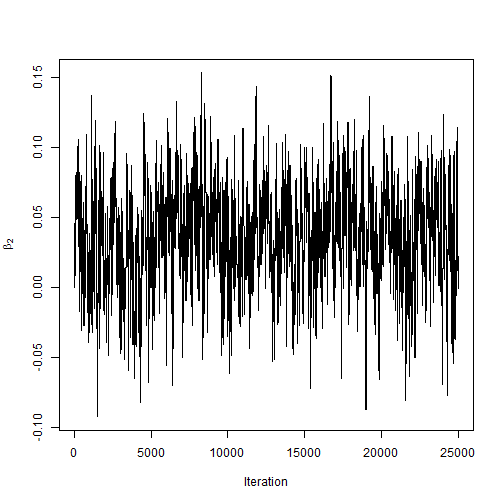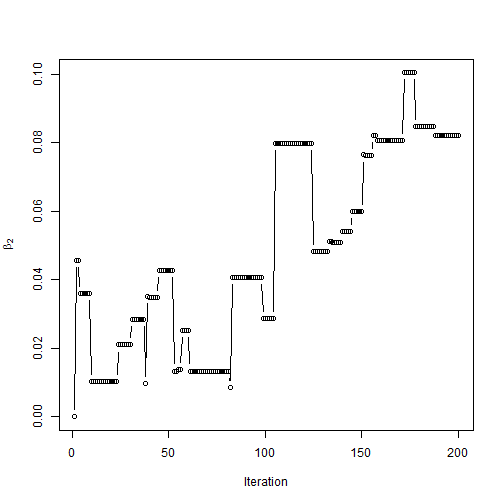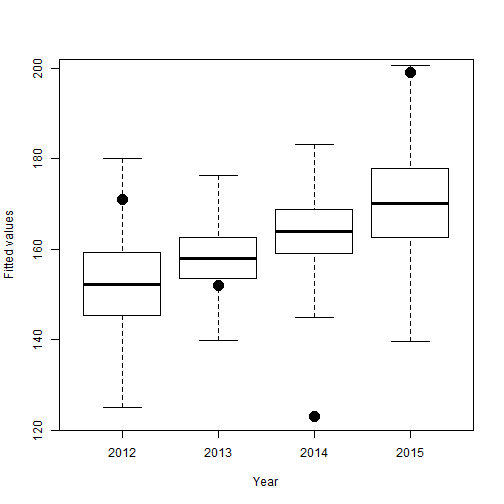# Metropolis sampling for the concussions data

### Chapter 3.2.3: Metropolis sampling

Let $$Y_i$$ be the number of concussions (aggregated over all teams and games) in season $$i$$ (1=2012,…,4=2015). We model these counts as $Y_i\sim\mbox{Poisson}(N\lambda_i) \mbox{ where } \lambda_i=\exp(\beta_1+i\beta_2),$ $$N$$ is the number of games played per year and $$\lambda_i$$ is the rate in year $$i$$. To complete the Bayesian model, we specify uninformative priors $$\beta_1,\beta_2\sim\mbox{Normal}(0,\tau^2)$$.

The log of the mean concussion rate is linear in time with $$\beta_2$$ determining the slope. The objective is to determine if the concussion rate is increasing, i.e., $$\beta_2>0$$.

 Y <- c(171, 152, 123, 199)
t <- 1:4
n <- 4
N <- 256


## Initialize

 # Create an empty matrix for the MCMC samples

S                 <- 25000
samples           <- matrix(NA,S,2)
colnames(samples) <- c("beta1","beta2")
fitted            <- matrix(0,S,4)

# Initial values

beta   <- c(log(mean(Y/N)),0)

# priors: beta[j] ~ N(0,tau^2)

tau    <- 10

# candidate standard deviations

can_sd <- rep(0.1,2)


## Define the log posterior as a function

 log_post <- function(Y,N,t,beta,tau){
mn    <- N*exp(beta+beta*t)
like  <- sum(dpois(Y,mn,log=TRUE))
prior <- sum(dnorm(beta,0,tau,log=TRUE))
post  <- like + prior
return(post)}


## Metropolis sampling

 for(s in 1:S){
for(j in 1:2){
can    <- beta
can[j] <- rnorm(1,beta[j],can_sd[j])
logR   <- log_post(Y,N,t,can,tau)-log_post(Y,N,t,beta,tau)
if(log(runif(1))<logR){
beta <- can
}
}
samples[s,] <- beta
fitted[s,]  <- N*exp(beta+beta*t)
}


## Compute the acceptance rates and plot the samples

 # Acceptance rates
colMeans(samples[1:24999,]!=samples[2:25000,])

##     beta1     beta2
## 0.4311772 0.1752070

 plot(samples[,1],type="l",xlab="Iteration",ylab=expression(beta))plot(samples[,2],type="l",xlab="Iteration",ylab=expression(beta))plot(samples[1:200,2],type="b",xlab="Iteration",ylab=expression(beta))## Summarize the fitted values for each year

The boxplots are the posterior distribution of the $$N\lambda_i=N\exp(\beta_1+i\beta_2)$$, and the points are the observed counts. The linear trend doesn't fit particularly well.

 boxplot(fitted,outline=FALSE,ylim=range(Y),
xlab="Year",ylab="Fitted values",names=2012:2015)
points(Y,pch=19,cex=2)# Posterior probability that the slope is positive
mean(samples[,2]>0)

##  0.84052


There is some evidence that the rate is increasing, but it seems to be driven only by the last year.